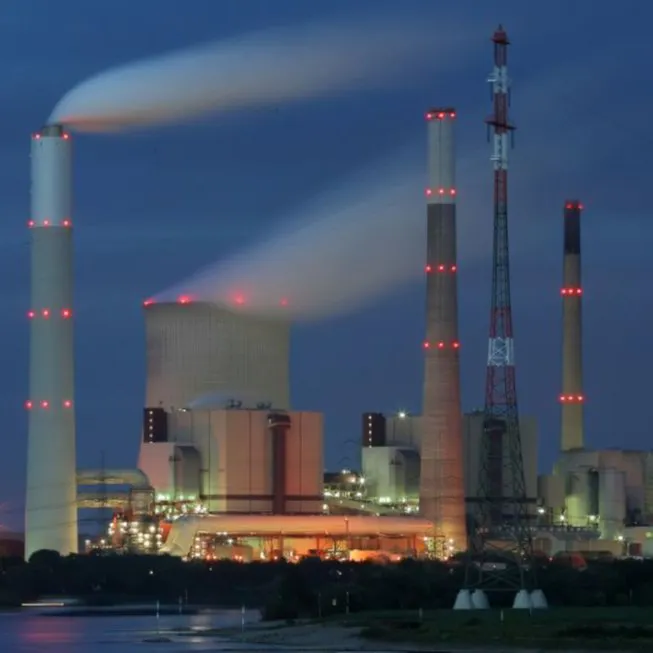# Project EEL

Monitoring river water quality based on open-design multi-parameter sonde, built along with QuickFeather and SensiML service.

IntermediateFull instructions providedOver 3 days609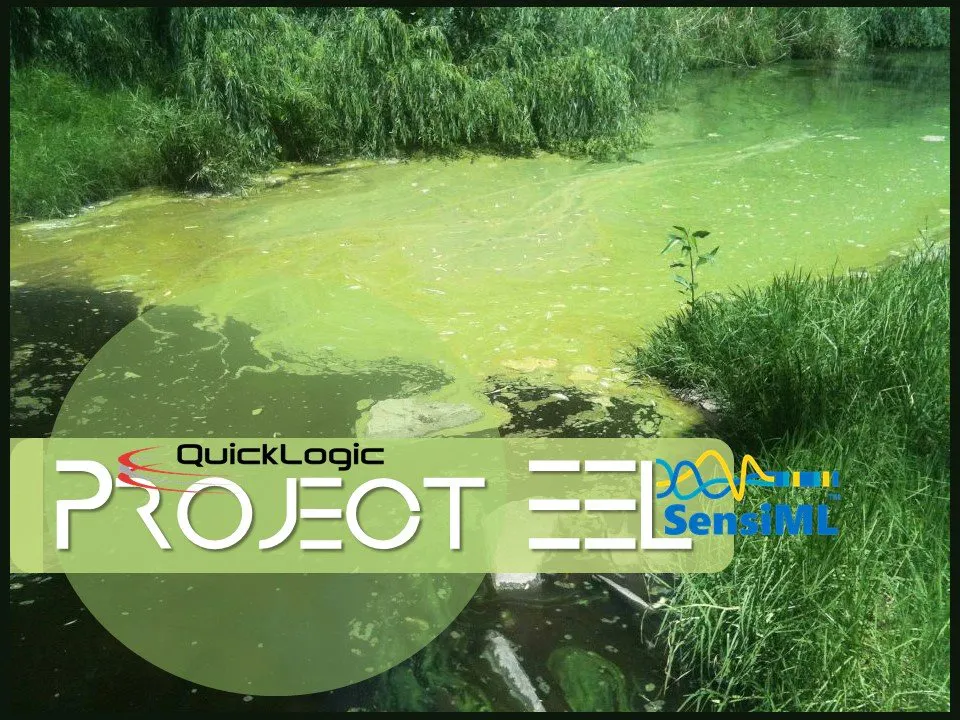## Things used in this project

### Hardware components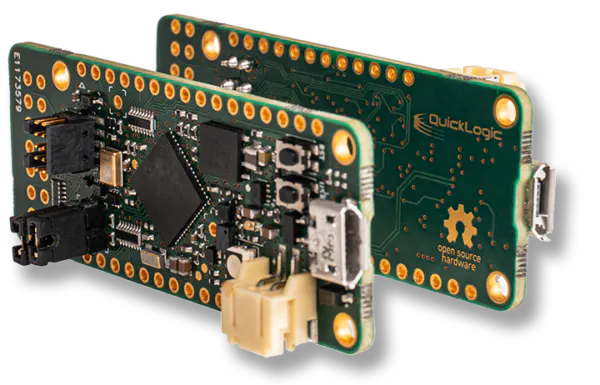QuickLogic Corp. QuickFeather Development Kit
×1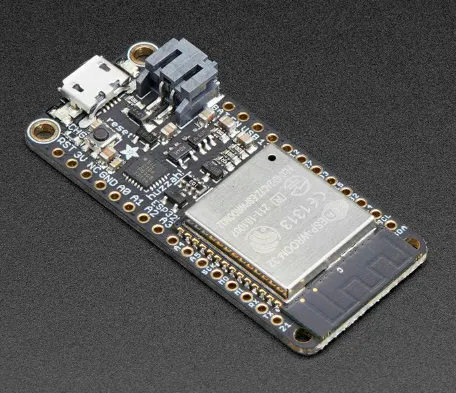Adafruit HUZZAH32 – ESP32 Feather Board
×1
 TinyCore32
×1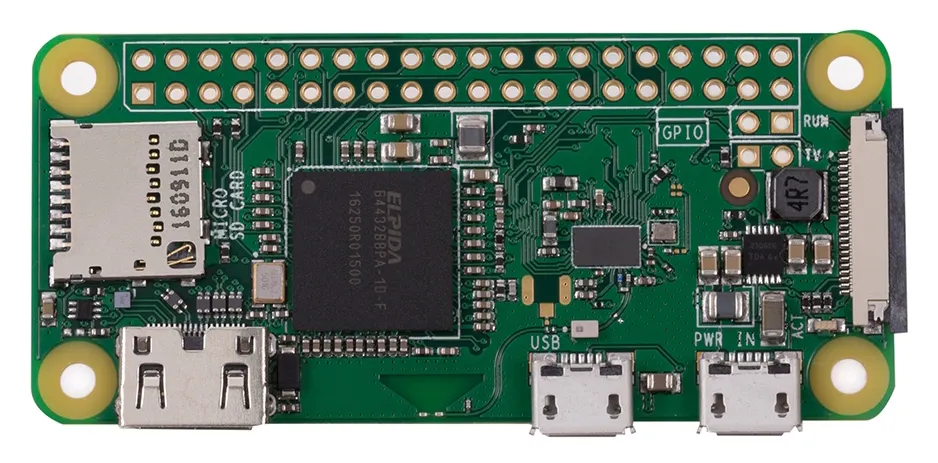Raspberry Pi Zero Wireless
×1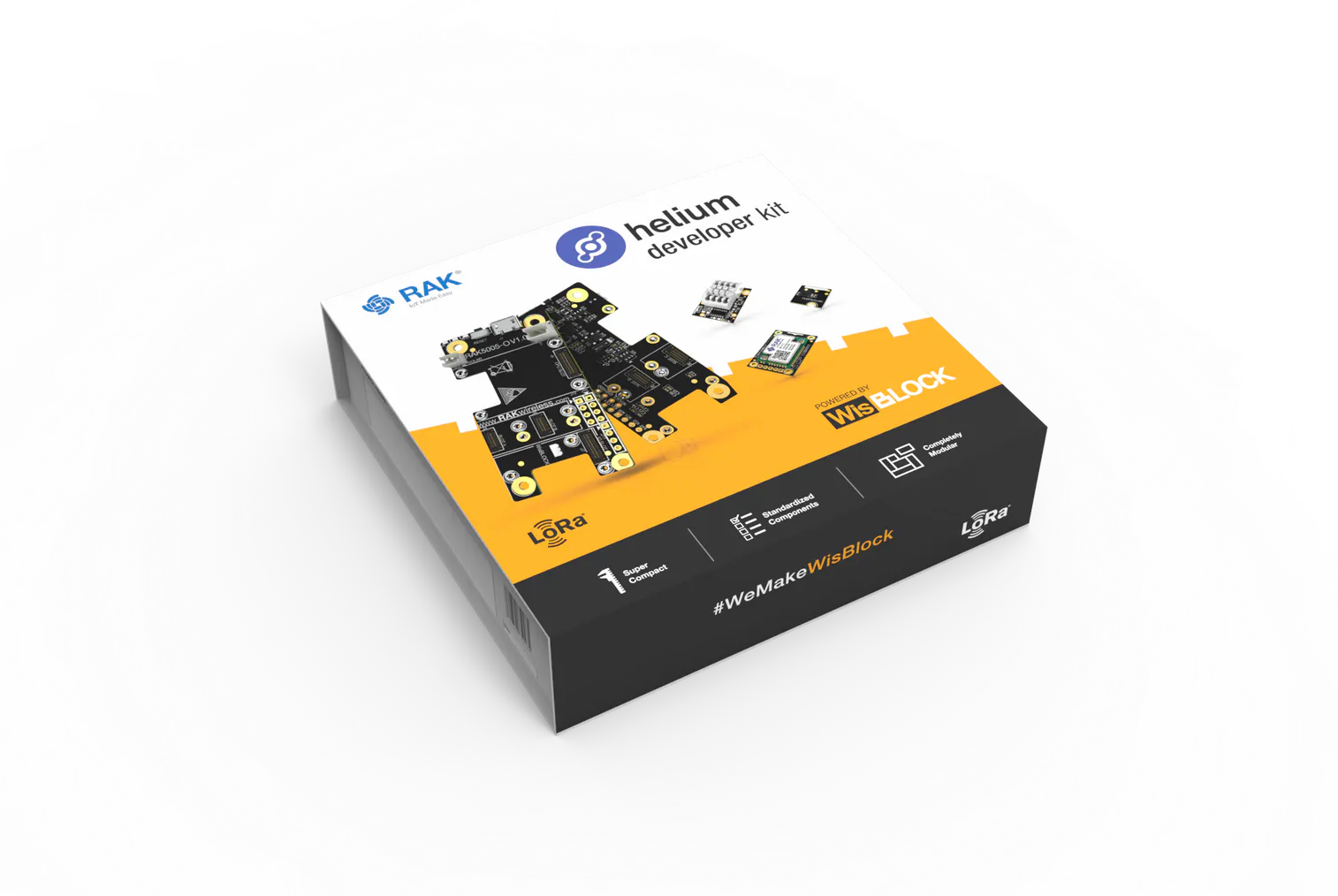Helium Developer Kit
×1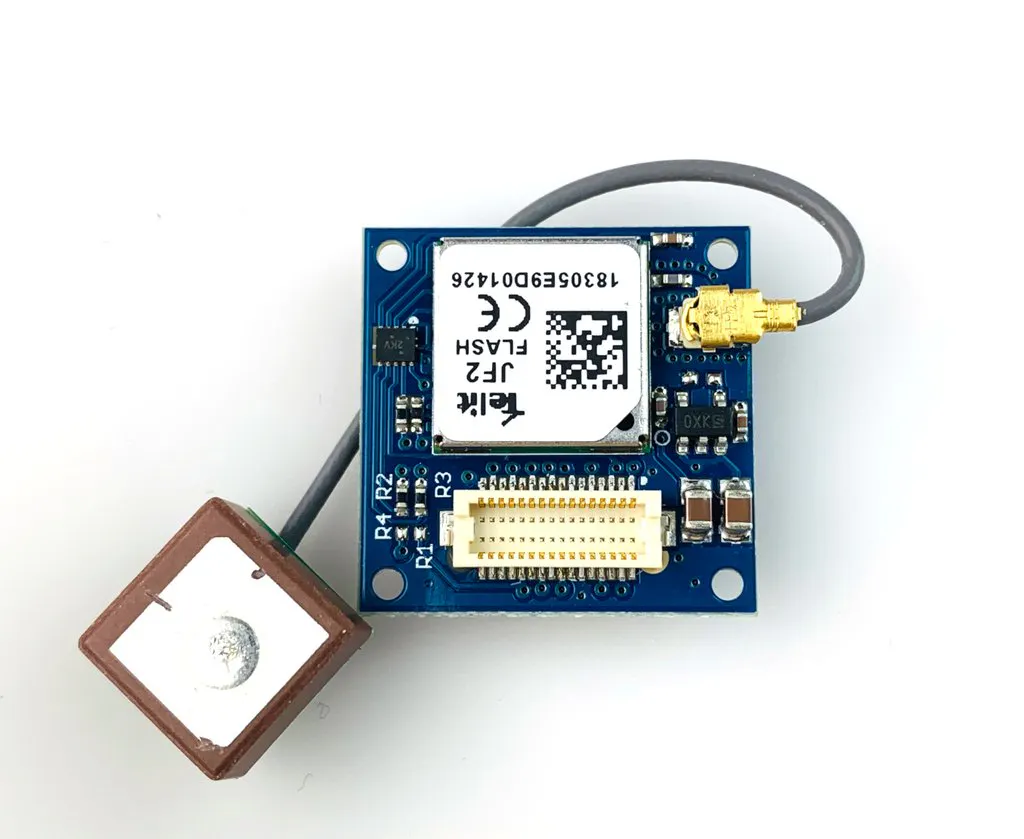TinyCircuits TinyShield GPS
×1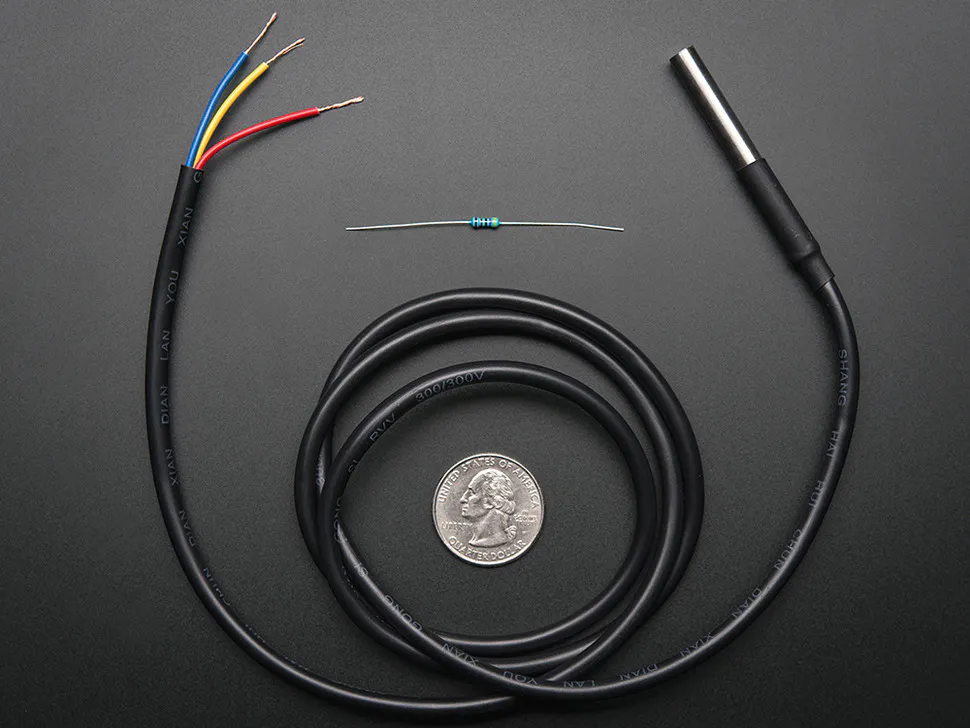Adafruit Waterproof DS18B20 Digital temperature sensor
×2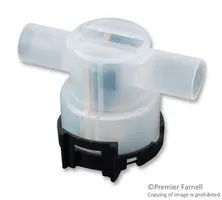Turbidity Sensor, Phototransistor Output
×1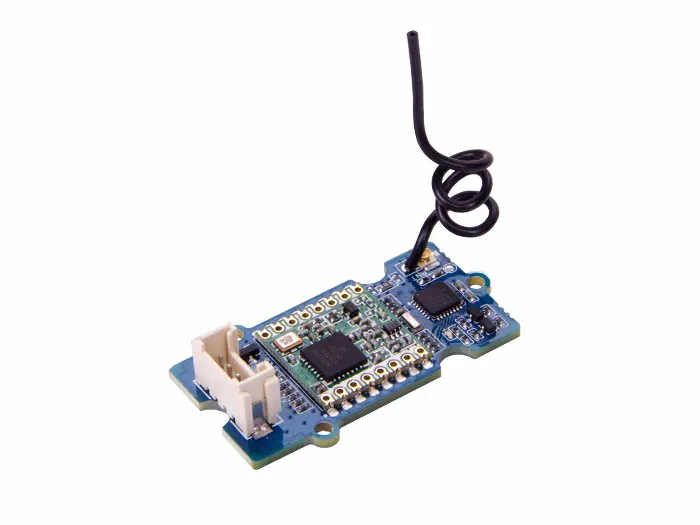Seeed Studio Grove - LoRa Radio 868MHz
×1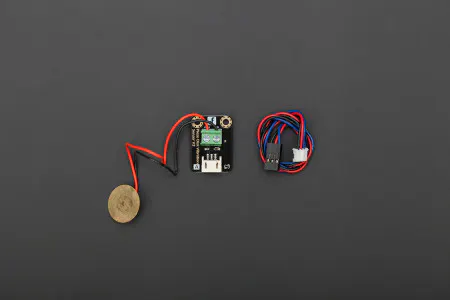DFRobot Gravity: Digital Piezo Disk Vibration Sensor
×1
 BMP280 Pressure sensor
×1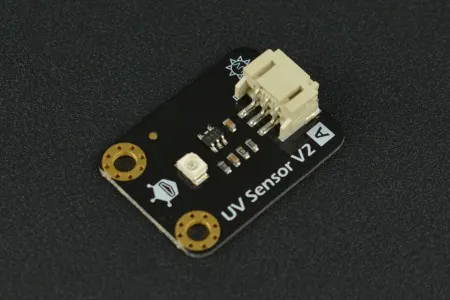DFRobot Gravity Analog UV Sensor V2
×1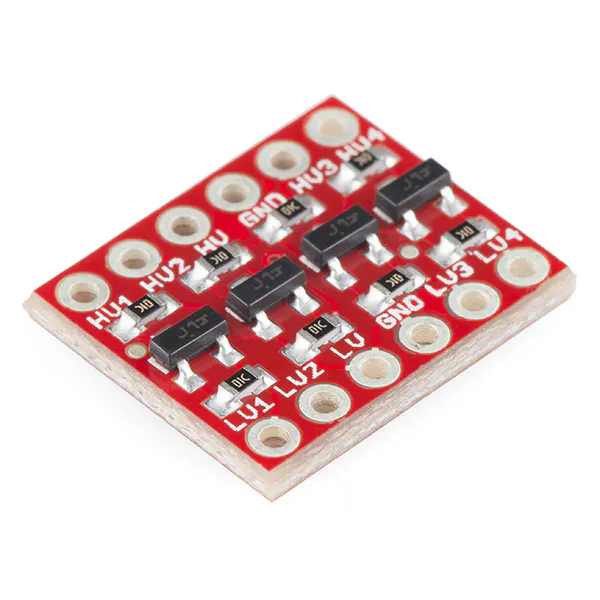SparkFun Logic Level Converter - Bi-Directional
×1
 UV LED & WS2812b
×1

### Software apps and online services

 QORC SDK
 SensiML Data Capture Lab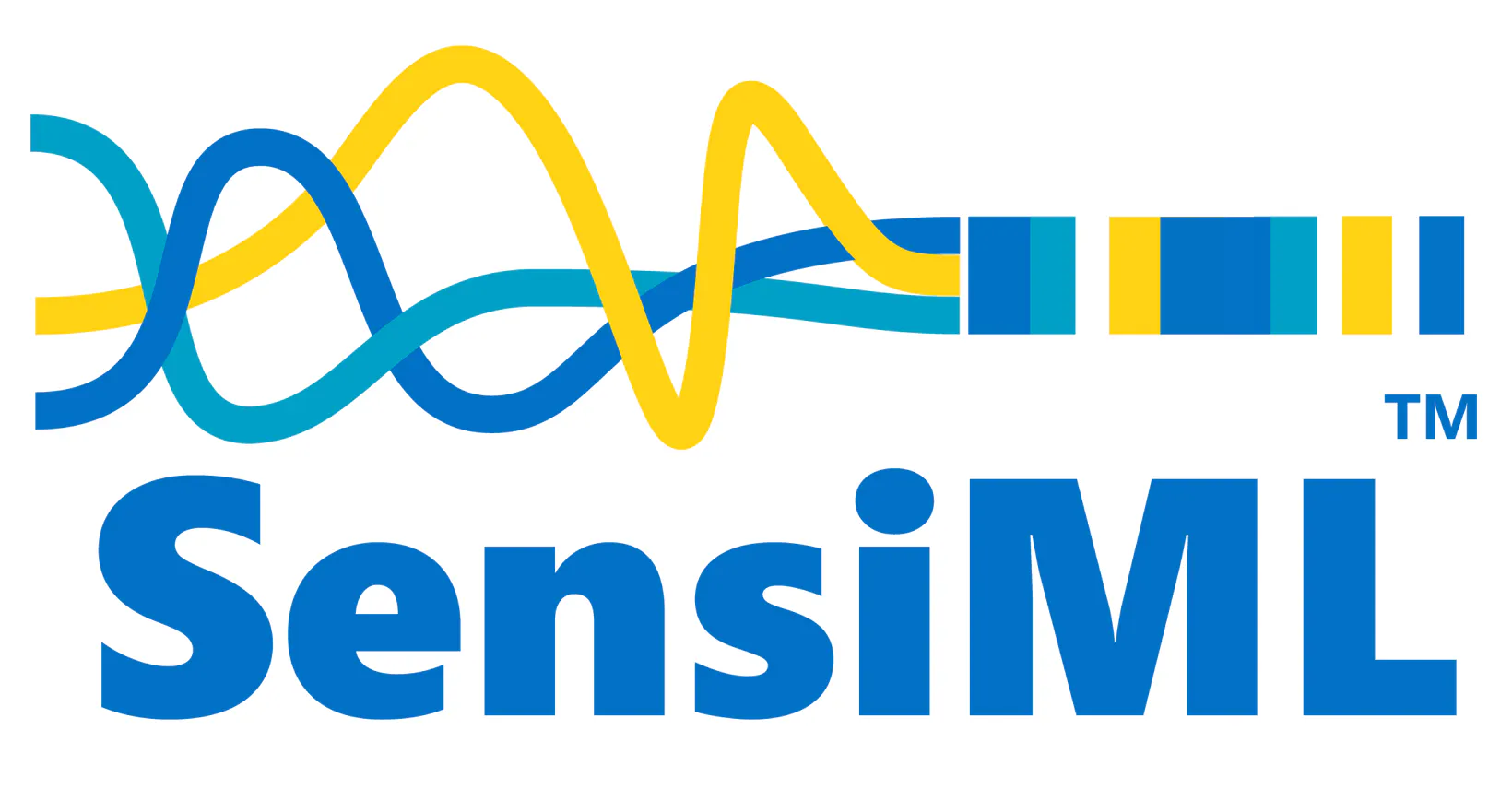SensiML Analytics Toolkit
 InfluxDB CloudArduino IDE

### Hand tools and fabrication machines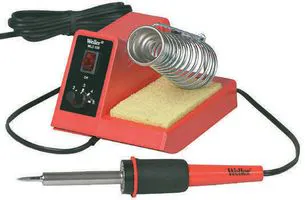Soldering Station, Hobbyist3D Printer (generic)Hot glue gun (generic)

## Schematics

### Hardware connection block diagram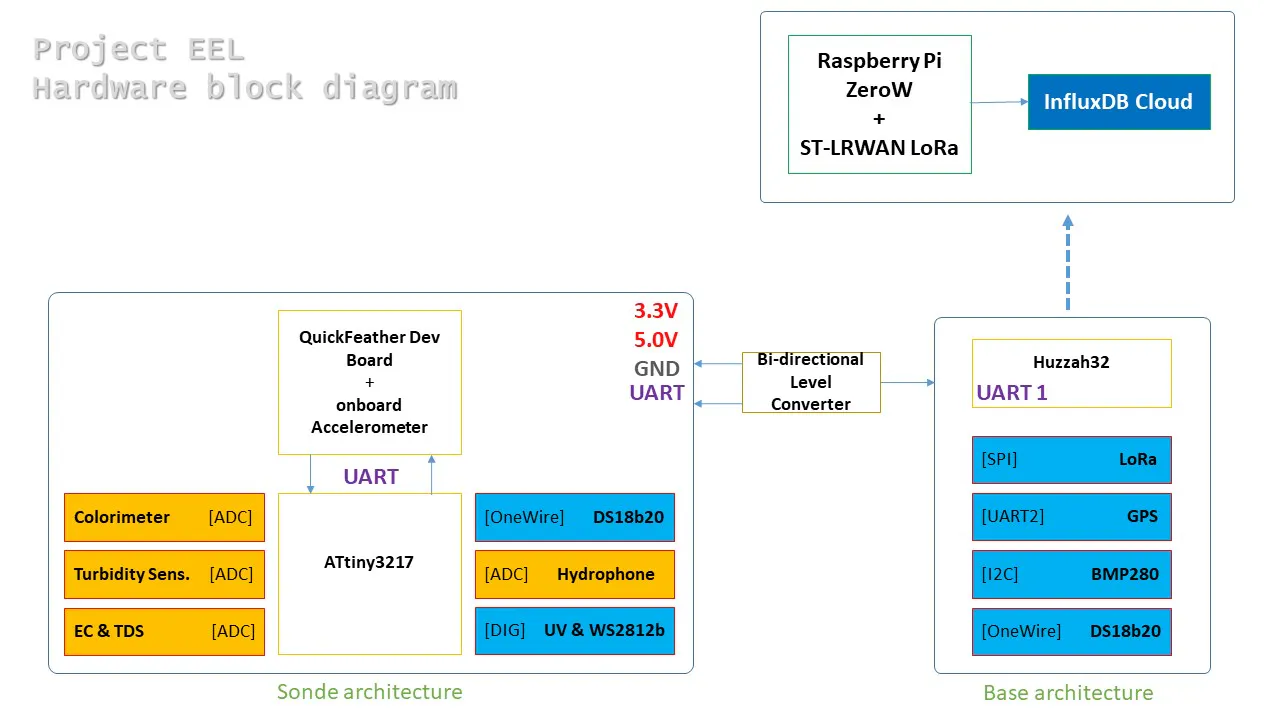## Code

### Sensor Data Router (BASE)

C/C++
```//Code for BASE unit that collects data from Sonde and sends to gateway over LoRa.

/*Demo Code for Project_EEL.
*GPS--Huzzah32(H32)
*DS18B20--H32
*BMP280--H32
*UART(H32)RX.--L.C.--TX.UART(ATtiny3217)
*UART(QF).TX--L.C.--RX.UART(ATtiny3217)
*/
//Test data added in Mk3 and Mk2.  Original Code Mk1.
#include <TinyGPS++.h>
#include <HardwareSerial.h>
#include <OneWire.h>
#include <DallasTemperature.h>
#include <Wire.h>
#include <SPI.h>
#include <SPI.h>
#include <LoRa.h>

#define ONE_WIRE_BUS 33     //Tested.
#define ss 4
#define rst 21
#define dio0 33
HardwareSerial tGPS(2);
#define gpsRXPIN 14
#define gpsTXPIN 32

HardwareSerial SensorBoard(1);
#define sbRXPIN 15
#define sbTXPIN 12

String readString,Count, EC, TDS, Temp, NTU, PMS, HPS, QLState;
int arr1, arr2, arr3, arr4, arr5, arr6, arr7, arr8;
char c;
int TDSI, FlowRate;
float ECF,TempF,NTUF,PMSF,HPSF;

OneWire oneWire(ONE_WIRE_BUS);
DallasTemperature sensors(&oneWire);

TinyGPSPlus gps;

void setup()
{
Serial.begin(115200);
sensors.begin();
LoRa.setPins(ss, rst, dio0);
LoRa.begin(867E6);
bmp.begin();
pinMode(LED_BUILTIN, OUTPUT);
tGPS.begin(9600, SERIAL_8N1, gpsRXPIN, gpsTXPIN);
SensorBoard.begin(115200, SERIAL_8N1, sbRXPIN, sbTXPIN);
}

void loop()
{
digitalWrite(LED_BUILTIN, LOW);
Serial.print("Surface Probe Temperature...");
sensors.requestTemperatures();
Serial.println(sensors.getTempCByIndex(0));
Serial.print(F("Rt: "));
Serial.println(" degC");
Serial.print(F("Ra: "));
Serial.println(" mtr.");
while (tGPS.available() > 0)
displayInfo();
Serial.println();
//delay(2000);
delay(5000);
GetSerial();
delay(500);

LoRa.beginPacket();
LoRa.println("Project_EEL ");
LoRa.print("Base: ");
LoRa.print("[D/T:");LoRa.print(gps.date.day());LoRa.print("/");LoRa.print(gps.date.month());LoRa.print("/");LoRa.print(gps.date.year());LoRa.print("  ");
LoRa.print(gps.time.hour());LoRa.print(":");LoRa.print(gps.time.minute());LoRa.print(":");LoRa.print(gps.time.second());LoRa.print("],[Pos:");
LoRa.print(gps.location.lat(), 4);LoRa.print("  ");LoRa.print(gps.location.lng(), 4);
LoRa.endPacket();
delay(500);
LoRa.beginPacket();
LoRa.print("SONDE: ");LoRa.print("[EC:");LoRa.print(ECF);LoRa.print("],[TDS:");LoRa.print(TDSI);LoRa.print("],[Temp:");LoRa.print(TempF);
LoRa.print("],[NTU:");LoRa.print(NTUF);LoRa.print("],[PMS:");LoRa.print(PMSF);LoRa.print("],[HPS:");LoRa.print(HPSF);LoRa.print("],[F.R.Sate:");LoRa.print(FlowRate);LoRa.print("]");
LoRa.endPacket();
digitalWrite(LED_BUILTIN, HIGH);
delay(2000);
}

void displayInfo()
{
Serial.print(F("Location: "));
if (gps.location.isValid())
{
Serial.print(gps.location.lat(), 4);
Serial.print(F(","));
Serial.print(gps.location.lng(), 4);
}
else
{
Serial.print(F("N/A"));
}

Serial.print(F("  Date/Time: "));
if (gps.date.isValid())
{
Serial.print(gps.date.month());
Serial.print(F("/"));
Serial.print(gps.date.day());
Serial.print(F("/"));
Serial.print(gps.date.year());
}
else
{
Serial.print(F("N/A"));
}

Serial.print(F(" "));
if (gps.time.isValid())
{
if (gps.time.hour() < 10) Serial.print(F("0"));
Serial.print(gps.time.hour());
Serial.print(F(":"));
if (gps.time.minute() < 10) Serial.print(F("0"));
Serial.print(gps.time.minute());
Serial.print(F(":"));
if (gps.time.second() < 10) Serial.print(F("0"));
Serial.print(gps.time.second());
}
else
{
Serial.print(F("N/A"));
}

Serial.println();
}

void GetSerial()
{
while (SensorBoard.available() > 0)
{
if (c=='*')
{
//Serial.println();
/* // Print String for De-bugging.
//Serial.print("Count: ");
Serial.println(Count);
Serial.print("EC: ");
Serial.println(EC);
Serial.print("TDS: ");
Serial.println(TDS);
Serial.print("Temp: ");
Serial.println(Temp);
Serial.print("NTU: ");
Serial.println(NTU);
Serial.print("PMS: ");
Serial.println(PMS);
Serial.print("HPS: ");
Serial.println(HPS);
Serial.print("FlowRate: ");
Serial.println(QLState);
Serial.println();
*/
//////Int&FloatConversion..
ECF = EC.toFloat();
TDSI = TDS.toInt();
TempF = Temp.toFloat();
NTUF = NTU.toFloat();
PMSF = PMS.toFloat();
HPSF = HPS.toFloat();
FlowRate = QLState.toInt(); // TODO.
//////PrintValues.
Serial.print("EC: ");
Serial.println(ECF);
Serial.print("TDS: ");
Serial.println(TDSI);
Serial.print("Temp: ");
Serial.println(TempF);

Serial.print("NTU: ");
Serial.println(NTUF);
Serial.print("PMS: ");
Serial.println(PMSF);
Serial.print("HPS: ");
Serial.println(HPSF);

Serial.print("Flow Rate State: ");
Serial.println(FlowRate);

//Flush individual string data.
Count="";
EC="";
TDS="";
Temp="";
NTU="";
PMS="";
HPS="";
QLState="";
}
else{
}
}
}
```

### End-Node (Sonde)

C/C++
```// Sonde code with sensor data collec&calib. over ATtiny3217

#include <OneWire.h>
OneWire  ds(10);        //SDA_AT3217
#include <tinyNeoPixel.h>
#define WS_PIN 11       //SCL_AT3217
#define UV_PIN 6        //PB5_AT3217
#define DG_PIN 7        //PB4_AT3217     ||    //RST_PIN 7
#define NUMPIXELS 1 //WS2812 dot.

char c;
int p_count = 0;
int R1 = 1000;//BurdR.
int Ra = 25; //Resistance(Internal)of MCU_pin
int Color_Sense = A1;      //[PA1]MOSI_AT3217
int NTU_Sense = A2;     //[PA2]MISO_AT3217
int Hydr_Sense = A3;    //[PA3]SCK_AT3217
int EC_Sink = A4;       //[PA4]SS_AT3217
int EC_Sense = A5;      //[PA5]VREF_AT3217
int EC_Source = A6;     //[PA6]DAC_AT3217

int QL_State;
float NTU, HPS, PMS;

//PPM_Conversion...//[USA]PPMconverion:0.5//[EU]PPMconversion:0.64//[AU/IND]PPMconversion:0.7//float PPMconversion=0.6;(Avg.)
float PPMconversion=0.7;
//float TemperatureCoef = 0.019; //changes as per electrolyte we are measuring
float TemperatureCoef = 0.08; //calibrated for brackish water with high salt content.
float K=2.88; //electrode constant(~2.9/~3.0)

//************ Temp Probe Related *********************************************//
float celsius;
float Temperature = 10;
float EC = 0;
float EC25 = 0;
int ppm = 0;
float raw = 0;
float Vin = 5; //[reading as per 5.0V and 1024 bit ADC resolution]
float Vdrop = 0;
float Rc = 0;
tinyNeoPixel pixels = tinyNeoPixel(NUMPIXELS, WS_PIN, NEO_GRB + NEO_KHZ800);
int delayval = 500;

void setup()
{
Serial.begin(115200);
pixels.begin();
pinMode(UV_PIN, OUTPUT);
pinMode(EC_Sense,INPUT);
pinMode(EC_Source,OUTPUT);//Setting pin for sourcing current
pinMode(EC_Sink,OUTPUT);//setting pin for sinking current
digitalWrite(EC_Sink,LOW);//We can leave the ground connected permanantly
delay(100);
R1 = (R1+Ra);// compensate for Power Pin Resistance

Serial.println("EEL Data Acquisition...");
//delay(10000);   //TODO.
//pinMode(RST_PIN,OUTPUT);    //TODO.
//digitalWrite(RST_PIN, LOW);  //TODO.
//Serial.println("Parameters Initialised.");  //TODO.
}

void loop()
{
delay(200);
GetQLState();
//delay(200);
GetEC();//dont call this more that 1/5 Hz [once every five seconds] or you will polarise electrolyte.
GetTurbidity();
GetHydro();
GetPM();

Serial.print("#");
Serial.print(p_count);
Serial.print(",");
Serial.print(EC25);
Serial.print(",");
Serial.print(ppm);
Serial.print(",");
Serial.print(Temperature);
Serial.print(",");
Serial.print(NTU);
Serial.print(",");
Serial.print(PMS);
Serial.print(",");
Serial.print(HPS);
Serial.print(",");
Serial.print(QL_State);
Serial.println("*");

p_count++;
delay(5000); //(should be greater than 5seconds to prevent electrode-error)
}

void GetQLState(){
while (Serial.available() > 0)
{
if (c=='}')
{
//Serial.println(s);
QL_State = s - '0';
//Serial.println(QL_State);

}
else{
}
}
}

void GetEC(){
GetTemp();
Temperature = 27.25;
//************Estimates Resistance of Electrolyte ****************//
digitalWrite(EC_Source,HIGH);
digitalWrite(EC_Source,LOW);
//***************** Converts to EC **************************//
Vdrop = (Vin*raw)/1024.0;
Rc = (Vdrop*R1)/(Vin-Vdrop);
Rc = Rc-Ra; //acounting for Digital Pin Resistance
EC = 1000/(Rc*K);
//*************Compensating For Temperaure********************//
//EC25 = EC/ (1+ TemperatureCoef*(Temperature-25.0));
EC25 = 0.5;
//ppm = (EC25)*(PPMconversion*1000);
ppm = 380;
}

void GetTemp()
{
byte i;
byte present = 0;
byte type_s;
byte data;
//float celsius;
ds.reset_search();
delay(250);
return;
}

for( i = 0; i < 8; i++) {
//Serial.write(' ');
}

return;
}

case 0x10:
//Serial.println("  Chip = DS18S20");  // or old DS1820
type_s = 1;
break;
case 0x28:
//Serial.println("  Chip = DS18B20");
type_s = 0;
break;
case 0x22:
//Serial.println("  Chip = DS1822");
type_s = 0;
break;
default:
//Serial.println("Device is not a DS18x20 family device.");
return;
}

ds.reset();
ds.write(0x44, 1);        // start conversion, with parasite power on at the end

delay(1000);     // maybe 750ms is enough, maybe not
// we might do a ds.depower() here, but the reset will take care of it.

present = ds.reset();
for ( i = 0; i < 9; i++) {           // we need 9 bytes
}
int16_t raw = (data << 8) | data;
if (type_s) {
raw = raw << 3; // 9 bit resolution default
if (data == 0x10) {
// "count remain" gives full 12 bit resolution
raw = (raw & 0xFFF0) + 12 - data;
}
} else {
byte cfg = (data & 0x60);
// at lower res, the low bits are undefined, so let's zero them
if (cfg == 0x00) raw = raw & ~7;  // 9 bit resolution, 93.75 ms
else if (cfg == 0x20) raw = raw & ~3; // 10 bit res, 187.5 ms
else if (cfg == 0x40) raw = raw & ~1; // 11 bit res, 375 ms
// default is 12 bit resolution, 750 ms conversion time
}
celsius = (float)raw / 16.0;
//Serial.print("Temperature: ");
//Serial.print(celsius);
//Serial.println(" *C");
}

void GetTurbidity()
{
float NTUvoltage  = 0.0;
for (int i=0;i<100;i++)
NTUvoltage = NTUvoltage/100;
NTUvoltage = round_to_dp(NTUvoltage, 1);
if (NTUvoltage<2.5)
{ NTU = 3000; }
else
{ NTU = -1120.4*square(NTUvoltage)+5742.3*NTUvoltage-4353.8; }  //Calib. Curve eqn. defined by DFRobot sensor page.
delay(100);
}

float round_to_dp(float val, int dec)
{
float multiplier=powf(10.0f,dec);
val=roundf(val*multiplier)/multiplier;
return val;
}

void GetPM()
{
float PMS = 0.0;
for (int i = 0; i < NUMPIXELS; i++) {
// pixels.Color takes RGB values, from 0,0,0 up to 255,255,255
pixels.setPixelColor(i, pixels.Color(0, 150, 0)); // Moderately bright green color.
pixels.show(); // This sends the updated pixel color to the hardware.
delay(delayval); // Delay for a period of time (in milliseconds).
}
delay(100);
digitalWrite(UV_PIN, HIGH);
digitalWrite(UV_PIN, LOW);
delay(500);
}

void GetHydro()
{
float HPS = 0.0;
for (int j=0;j<100;j++)
HPS = HPS/100;
delay(500);
}
```

### LoRa Gateway

C/C++
```//ST-LRWAN based LoRa Receiver[Project EEL]:: connected to RPi0W.

void setup( void )
{
Serial.begin(115200);
while (!Serial) { }
}

void loop( void )
{
if (packetSize) {
}
Serial.print(", SNR: ");
Serial.println(")");
}
}
```

## Credits

### Night R

16 projects • 41 followers
R&D Engineer @ IoT Solutions Provider, Robotics Engineer @ SIS Corp., Passionate for Hardware hacking, 12+ years experience in programming..

### Shivam Kumar Tiwari

1 project • 4 followers
1. Currently Working as Junior Engineer (C& I) at UPRVUNL 2.Worked as PCB Design Engineer for 6Y At ACME DIGITEK SOLUTION PVT LTD.